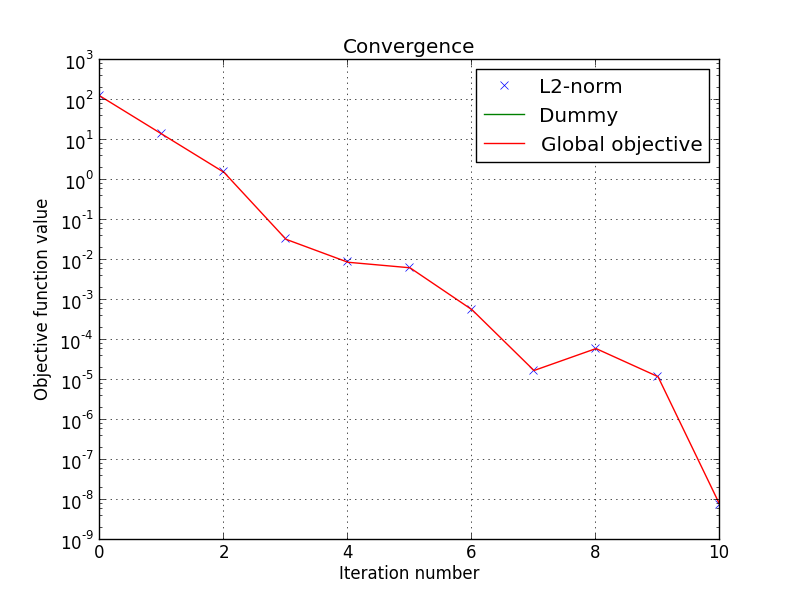# Simple least square problem¶

This simplistic example is only meant to demonstrate the basic workflow of the toolbox. Here we want to solve a least square problem, i.e. we want the solution to converge to the original signal without any constraint. Lets define this signal by :

>>> y = [4, 5, 6, 7]


The first function to minimize is the sum of squared distances between the current signal x and the original y. For this purpose, we instantiate an L2-norm object :

>>> from pyunlocbox import functions
>>> f1 = functions.norm_l2(y=y)


This standard function object provides the eval(), grad() and prox() methods that will be useful to the solver. We can evaluate them at any given point :

>>> f1.eval([0, 0, 0, 0])
126
array([ -8, -10, -12, -14])
>>> f1.prox([0, 0, 0, 0], 1)
array([ 2.66666667,  3.33333333,  4.        ,  4.66666667])


We need a second function to minimize, which usually describes a constraint. As we have no constraint, we just define a dummy function object by hand. We have to define the _eval() and _grad() methods as the solver we will use requires it :

>>> f2 = functions.func()
>>> f2._eval = lambda x: 0
>>> f2._grad = lambda x: 0


Note

We could also have used the pyunlocbox.functions.dummy function object.

We can now instantiate the solver object :

>>> from pyunlocbox import solvers
>>> solver = solvers.forward_backward()


And finally solve the problem :

>>> x0 = [0, 0, 0, 0]
>>> ret = solvers.solve([f2, f1], x0, solver, absTol=1e-5, verbosity='high')
INFO: Forward-backward method : FISTA
Iteration   1 : objective = 1.40e+01, relative = 8.00e+00
Iteration   2 : objective = 1.56e+00, relative = 8.00e+00
Iteration   3 : objective = 3.29e-02, relative = 4.62e+01
Iteration   4 : objective = 8.78e-03, relative = 2.75e+00
Iteration   5 : objective = 6.39e-03, relative = 3.74e-01
Iteration   6 : objective = 5.71e-04, relative = 1.02e+01
Iteration   7 : objective = 1.73e-05, relative = 3.21e+01
Iteration   8 : objective = 6.11e-05, relative = 7.17e-01
Iteration   9 : objective = 1.21e-05, relative = 4.04e+00
Iteration  10 : objective = 7.46e-09, relative = 1.62e+03
Solution found after 10 iterations :
objective function f(sol) = 7.460428e-09
last relative objective improvement : 1.624424e+03
stopping criterion : ABS_TOL


The solving function returns several values, one is the found solution :

>>> ret['sol']
array([ 3.99996922,  4.99996153,  5.99995383,  6.99994614])


Another one is the value returned by each function objects at each iteration. As we passed two function objects (L2-norm and dummy), the objective is a 2 by 11 (10 iterations plus the evaluation at x0) ndarray. Lets plot a convergence graph out of it :

>>> import numpy as np
>>> import matplotlib, sys
>>> cmd_backend = 'matplotlib.use("AGG")'
>>> _ = eval(cmd_backend) if 'matplotlib.pyplot' not in sys.modules else 0
>>> import matplotlib.pyplot as plt
>>> objective = np.array(ret['objective'])
>>> _ = plt.figure()
>>> _ = plt.semilogy(objective[:, 1], 'x', label='L2-norm')
>>> _ = plt.semilogy(objective[:, 0], label='Dummy')
>>> _ = plt.semilogy(np.sum(objective, axis=1), label='Global objective')
>>> _ = plt.grid(True)
>>> _ = plt.title('Convergence')
>>> _ = plt.legend(numpoints=1)
>>> _ = plt.xlabel('Iteration number')
>>> _ = plt.ylabel('Objective function value')
>>> _ = plt.savefig('doc/tutorials/simple_convergence.pdf')
>>> _ = plt.savefig('doc/tutorials/simple_convergence.png')


The below graph shows an exponential convergence of the objective function. The global objective is obviously only composed of the L2-norm as the dummy function object was defined to always evaluate to 0 (f2._eval = lambda x: 0).